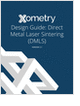MaterialsDesign CenterProcessesUnit ConversionFormulasMathematicsCalculatorsDiscussion ForumTrade PublicationsDirectory Service
Salary Expectation

8 things to know about the interview question "What's your salary expectation"?

CNC Machining Design Guide

Learn the best principles to negotiate the salary you deserve!

Metal 3D Printing Design Guide

Direct Metal Laser Sintering (DMLS) 3D printing for parts with reduced cost and little waste.

more free magazinesGlossary » Units » Moment, Torque » Newton-CentimeterNewton-Centimeter (N-cm) is a unit in the category of Moment, Torque. It is also known as centimetres, cm-N, centimeters. This unit is commonly used in the SI unit system. Newton-Centimeter (N-cm) has a dimension of ML2T-2 where M is mass, L is length, and T is time. It can be converted to the corresponding standard SI unit N-m by multiplying its value by a factor of 0.01.
Note that the seven base dimensions are M (Mass), L (Length), T (Time), Q (Temperature), N (Aamount of Substance), I (Electric Current), and J (Luminous Intensity).

Other units in the category of Moment, Torque include Dyne-Centimeter (dyn-cm), Gram Force-Centimeter (gf-cm), Kilogram Force-Meter (kgf-m), Newton-Meter (N-m), Ounce Force-Inch (ozf-in), Pound Force-Foot (lbf-ft), Pound Force-Inch (lbf-in), and Poundal-Foot (pdl-ft).N/ARelated PagesSalary Expectation

8 things to know about the interview question "What's your salary expectation"?CNC Machining Design Guide